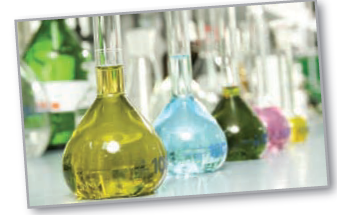Chapter 8.5, Problem 50E

Chapter
Section
Textbook Problem

# Chemical Reaction In a chemical reaction, one unit of compound Y and one unit of compound Z are converted into a single unit of compound X. Let x be the amount of compound X formed. The rate of formation of X is proportional to the product of the amounts of unconverted compounds Y and Z. So, d x / d t = k ( y 0 − x ) ( z 0 − x ) , where y 0 and z 0 are the initial amounts of compounds Y and Z. From this equation, you obtain∫ 1 ( y 0 − x ) ( z 0 − x ) d x = ∫ k   d t . (a) Solve for x as a function of t.(b) Use the result of part (a) to find x as t → ∞ for ( 1 ) y 0 < z 0 ,   ( 2 ) y 0 > z 0 , and   ( 3 ) y 0 = z 0 .

(a)

To determine

To calculate: The value of x as a function of t.

Explanation

Given:

dxdt=k(y0x)(z0x)1(y0x)(z0x)dx=kdt

Formula used:

1(y0x)(z0x)dx=kdt

Calculation:

Consider the provided function,

1(y0x)(z0x)dx=kdt …...…...…...(1)

Now, take LHS of equation (1),

1(y0x)(z0x)dx

By using partial fraction method we get,

1(y0x)(z0x)=A(y0x)+B(z0x)1(y0x)(z0x)=A(z0x)+B(y0x)(y0x)(z0x)

By simplifying further, we get,

1=A(z0x)+B(y0x)

Substitute em x=/em y0 and emx=/em z0 in above equation,

Simplify to get,

A=B=1(z0y0)

Now substitute values of A and B

1(y0x)(z0x)=A(y0x)+B(z0x)=1/(z0y0)(y0x)+1/(z0y0)(z0x)=1(z0y0)(y0x)1(z0y0)(z0x)

Now on simplification we get the integral as follows,

1(y0x)(z0x)dx=1(z0y0)(1(y0x)1(z0x))dx

(b)

To determine

To calculate: The value of x as t for y0<z0, y0>z0 and y0=z0

### Still sussing out bartleby?

Check out a sample textbook solution.

See a sample solution

#### The Solution to Your Study Problems

Bartleby provides explanations to thousands of textbook problems written by our experts, many with advanced degrees!

Get Started

#### Differentiate the function. y = ex + 1 + 1

Single Variable Calculus: Early Transcendentals, Volume I

#### Fill in the blanks. 8. a. The general form of an equation of a line is_______.

Applied Calculus for the Managerial, Life, and Social Sciences: A Brief Approach

#### CHECK POINT True or false: 4.

Mathematical Applications for the Management, Life, and Social Sciences